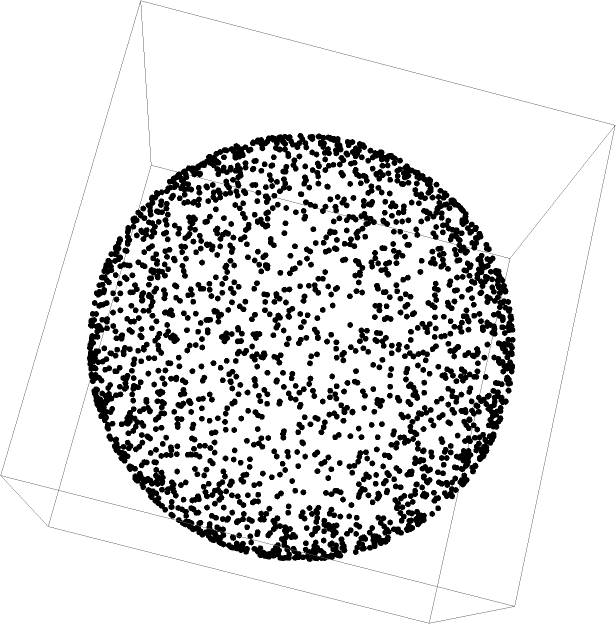Function Repository Resource:

RandomRotationQuaternion

Return uniformly distributed random rotations in quaternion form

Contributed by: Sander Huisman
 ResourceFunction["RandomRotationQuaternion"][] returns a unit quaternion from uniformly distributed random rotations. ResourceFunction["RandomRotationQuaternion"][m] returns m unit quaternions from uniformly distributed random rotations.

Details

The rotation axis is chosen randomly from a unit sphere. Rotations around that axis have to be chosen from a specific distribution to uniformly generate random orientations, see Ref. .

Examples

Basic Examples (1)

Generate a random orientation:

 In:=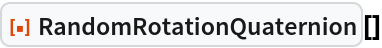Out=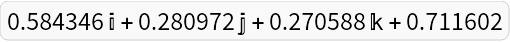Scope (1)

Generate 5 random orientations:

 In:=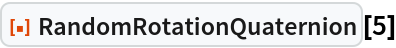Out=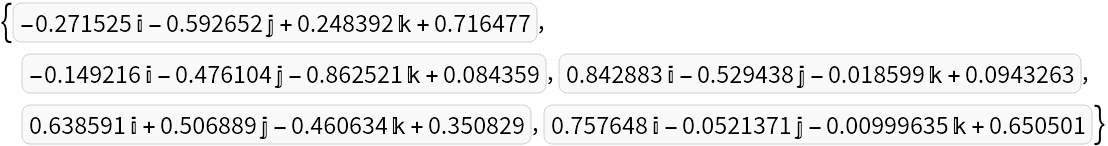Properties and Relations (1)

The norm of a quaternion corresponding to random orientations is equal to 1:

 In:=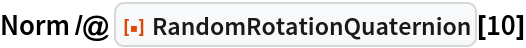Out=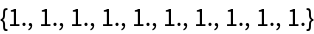Neat Examples (1)

Generate 2500 random points on a sphere by rotating the vector {0,0,1} with random orientations:

 In:=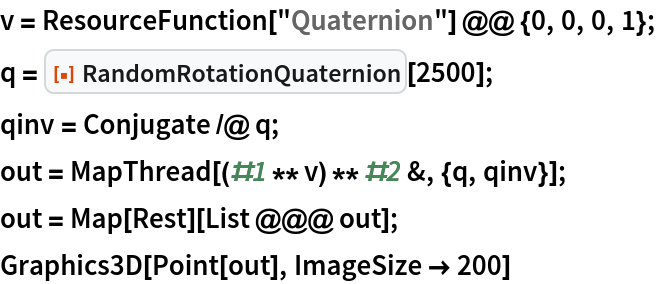Out=